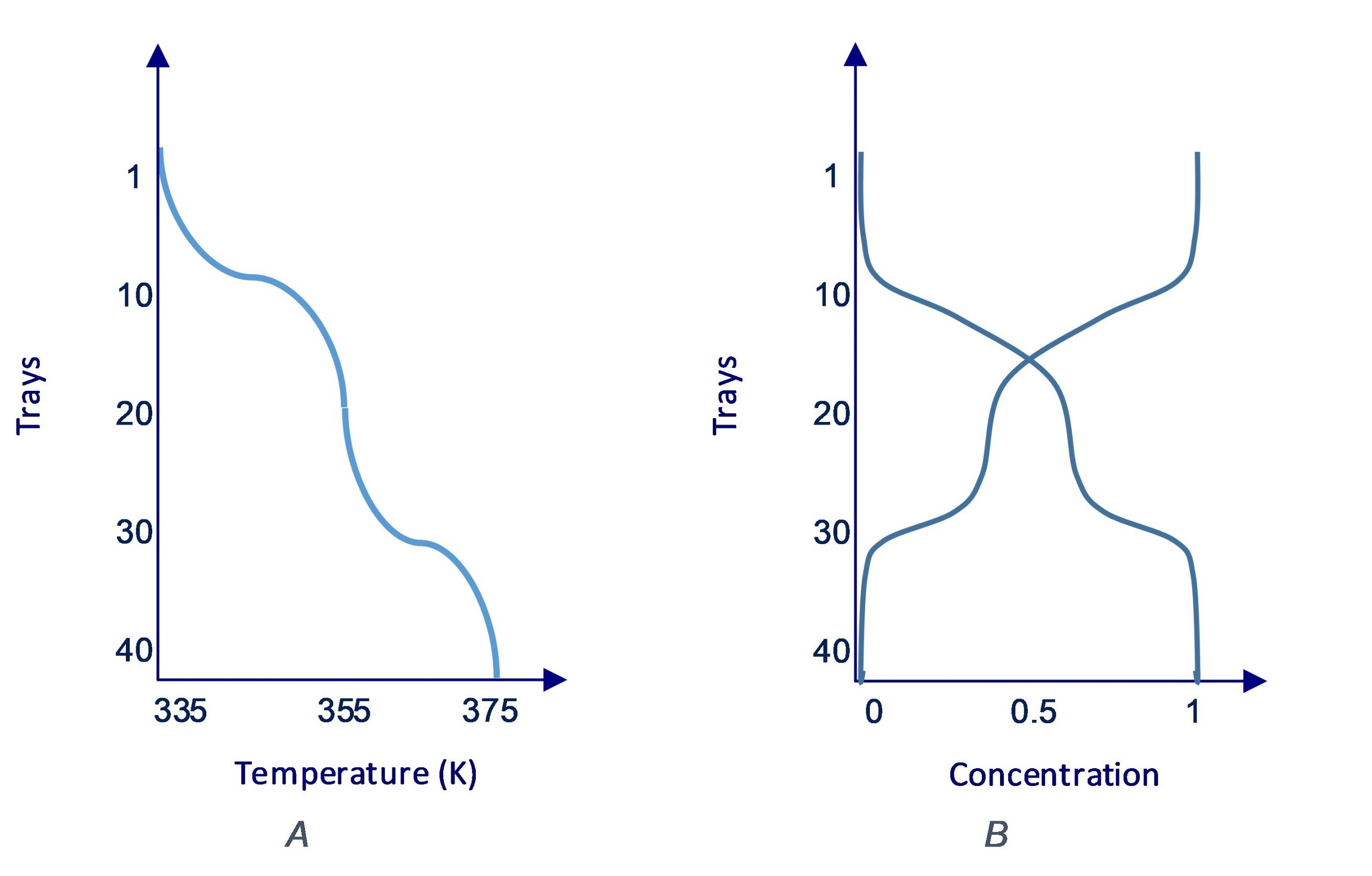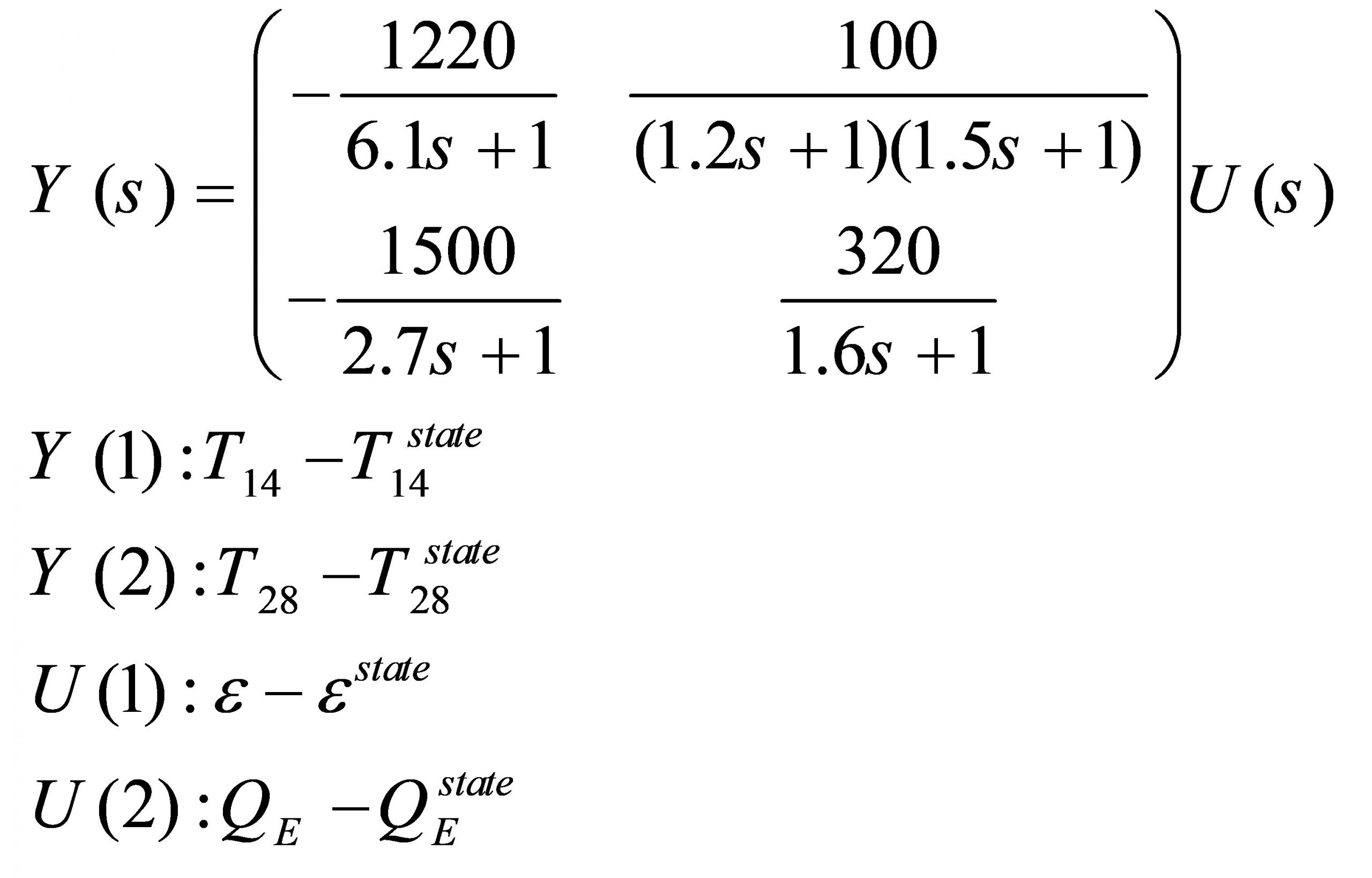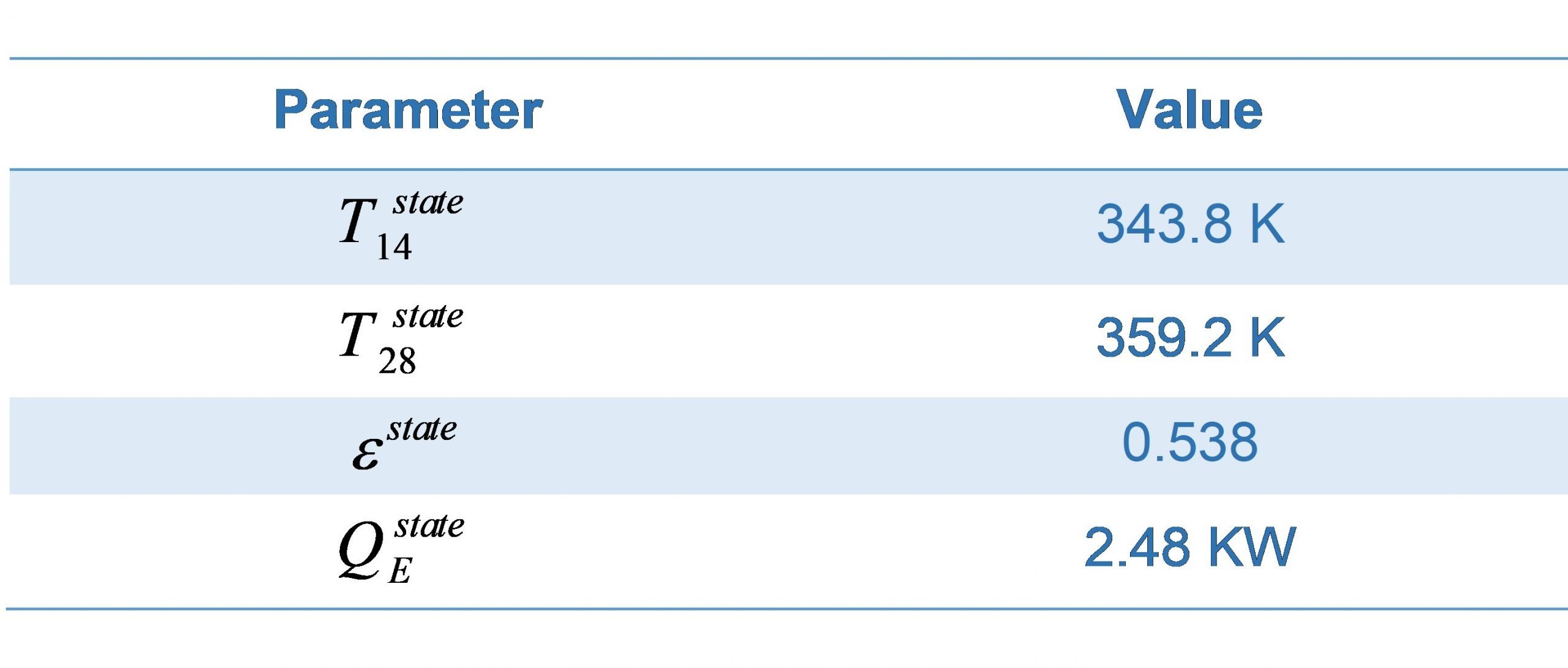# 11 Distillation column system

Multi-input multi-output systems have a very important place in the subject of control systems, because many real systems have multiple inputs and affect multiple outputs at the same time. One of the best examples of this type of systems is the distillation column system used in chemical industry to separate two materials with different boiling points from each other.

You can see a simple model of the distillation system in Figure 6.1. Mixed material (feed) is heated at the bottom of the column using the re-boiler (QE) and rises after evaporation. Inside the tower, there are 40 trays with different temperatures that collect the material with the appropriate boiling point. The system has a condenser at the top of the column which turns the steam to liquid and refluxes some of it back in the system according to the reflux ratio (ε ). A binary distillation column separates two materials from each other where the outputs are the concentration of them in the 1st and 40th trays.

In this system, the feed is considered as a disturbance because there is no control on it. To solve the system and find the relationship between the outputs and the inputs of this system analytically, several linear and non-linear differential equations must be solved. However, we can use a trick to simplify the problem. The easy method is to only consider the temperature of trays 14 and 28 as outputs so that the system is approximately in the desired temperature state (Figure 6.2–a). In this case, the system has the best performance and in trays 1 and 40, the two materials are pure (Figure 6.2–b).Figure 6.2–a) desired temperature state b) concentration in different trays in desired temperature state

Using this feature and doing some simplification like linearization we can have the following relationship between the outputs and inputs:The values of steady state parameters are given in Table 6.1.

Table 1–values of steady state parametersObjectives

The following objectives will be covered as you go through the experiment procedure.

• Derive the state space model of a transfer function system.
• Determine the characteristic polynomial, roots and modes of a system from its state space.
• Become familiar with the transition matrix
• Determine the system response to initial condition, impulse and other inputs in state space
• Discuss the internal and external stability in state space.

Procedure

1. Derive the canonical controllable state space of the system.

2. According to your knowledge from previous experiments what is the disturbance to the system?

3. Solve the following questions theoretically and then verify your answers in MATLAB.

a) Derive the characteristic polynomial, roots, modes and transition matrix of the system according to Step 1?

b) Imagine that the tray temperature is [T14   T28]= [353.8  349.2] , what’ll happen if the re-boiler temperature and reflux ratio stay in ideal state (zero-input response of the system)?

c) What will happen if a large amount of reflux, suddenly pours into the column? Note that the re-boiler works with gas. What will happen if an unpredicted spark led to a small explosion in the re-boiler (Unit impulse response of the system)?

d) If all trays in the column are in ideal state and the re-boiler warms up following this equation ε(t)=εstate (1.1-0.1e-0.1t)u(t) what will be the output (zero-state response of the system)?

e) What is the output if in part d if all trays’ temperature were in 300 kelvins initially (total response of the system)?

4. Is this system BIBO-stable?

5. Is this system internally stable? Why?

6. What’s the difference between BIBO-stability in MIMO and SISO systems?